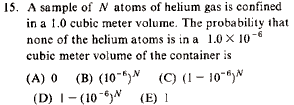GR 8677927796770177 | # Login | Register

GR8677 #15
Problem
 GREPhysics.NET Official Solution Alternate SolutionsStatistical Mechanics}Probability

Probability is mostly common sense and adhering to definitions.

The probability that a gas molecule or atom is in the small cube is . The probability that it's not in that small cube is . Assuming independent gas molecules or atoms, i.e., the usual assumption of randomness in Stat Mech, one gets, .Alternate Solutions
 There are no Alternate Solutions for this problem. Be the first to post one!
ernest21
2019-08-10 03:09:28
A great day and a very explicit information. apex hq
 danielsw986672019-10-21 05:20:36 With Kinetic Theory and the atomic and molecular explanation of pressure, the answer is c. business for sale cincinnati ohiofredluis
2019-08-08 13:02:41
The writing style is somewhat conversational and straightforward, and the problems show a nice range of difficulty. tile contractorjoshuaprice153
2019-08-08 07:04:25
I was suggested this blog by means of my bro. I am not sure whether or not this publish is written by means of him as no one else understand such precise about my problem. You’re wonderful! Thanks! towing serviceyosun2015tester
2015-07-22 12:26:56
whatyosun2015tester
2015-07-22 12:25:20
testing123 july 22 2015mpdude8
2012-04-15 20:09:22
For this, I just looked at it logically. Think about N = a small number, like 2. A and E are out -- there's always at least a chance that neither one will be in that subset, or one of them will. Also, when N = 2, the probability that both particles are outside of such a small subset of the total volume should be almost 1. B is out, as even for N = 2, the probability is extremely small, and races towards zero as you increase N slightly.

D is also out, as it is the exact opposite effect of what you want, logically. Probability, as you add more particles, should tend to 0. C is the only choice that has the correct tendency as N grows, and at a reasonable rate of increase.livieratos
2011-11-07 03:54:30
so the probability of one atom to be in the given small volume is the ratio of the small volume to the entire volume? P(yes) = 1E-6/1 = 1E-6?
 Ajith2015-10-14 09:30:40 I guess \r\nHere, No. of atoms in = N\r\nNo. of atoms in = No. of atoms in =\r\nThus, =ubaraj
2007-11-01 01:15:13
the volume is 1. There can be no He atoms in the volume 10E-6. So all N atoms have to be within the volume (1-10E-6), and so the probability becomes (1-10E-6)^N. The choice is C!!Furious
2007-08-17 15:40:58
You really just need to look at the limiting factors in this problem.

When N is 0 the probability should be 1. That eliminates A and D.

When N is extremely high, the probability should go to 0. That eliminates E, (not that any of us thought it was E)

So that leaves us with B and C. Which both match the limiting cases, but just think about it when N is 1. When N is one there should be a very high chance that it is not in the specific 1e-6 area.

For B, the probability is 1e-6, not very high at all.
For C, the probability is 0.99999, pretty close to 1.

This helped me since, I get super confused whenever I think about probability.wishIwasaphysicist
2006-01-24 11:27:10
How did you connect P(Ngasatoms) = (1-1E-6)^N to answer C?
 yosun2006-02-01 22:02:05 hi wishIwasaphysicist, because each of the N molecules are independent, the probability would be multiplicative. Thus, if the particle for each particle is P, the probability for N particles would be .
 yosun2015tester2015-07-22 12:31:28 testing123
 yosun2015tester2015-07-22 12:37:21 testering12345
 yosun2015tester2015-07-22 12:49:08LaTeX syntax supported through dollar sign wrappers $, ex.,$\alpha^2_0$produces . type this... to get...$\int_0^\infty\partial\Rightarrow\ddot{x},\dot{x}\sqrt{z}\langle my \rangle\left( abacadabra \right)_{me}\vec{E}\frac{a}{b}\$